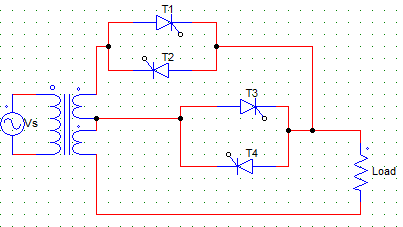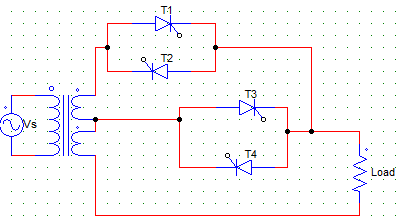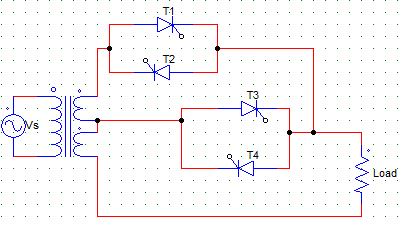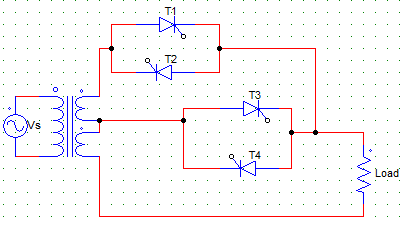Test: Sequence Controller

# Test: Sequence Controller

Test Description

## 10 Questions MCQ Test Power Electronics | Test: Sequence Controller

Test: Sequence Controller for Electrical Engineering (EE) 2023 is part of Power Electronics preparation. The Test: Sequence Controller questions and answers have been prepared according to the Electrical Engineering (EE) exam syllabus.The Test: Sequence Controller MCQs are made for Electrical Engineering (EE) 2023 Exam. Find important definitions, questions, notes, meanings, examples, exercises, MCQs and online tests for Test: Sequence Controller below.
Solutions of Test: Sequence Controller questions in English are available as part of our Power Electronics for Electrical Engineering (EE) & Test: Sequence Controller solutions in Hindi for Power Electronics course. Download more important topics, notes, lectures and mock test series for Electrical Engineering (EE) Exam by signing up for free. Attempt Test: Sequence Controller | 10 questions in 10 minutes | Mock test for Electrical Engineering (EE) preparation | Free important questions MCQ to study Power Electronics for Electrical Engineering (EE) Exam | Download free PDF with solutions
 1 Crore+ students have signed up on EduRev. Have you?
Test: Sequence Controller - Question 1

### Sequence control of ac voltage controllers is employed for the improvement of _________

Detailed Solution for Test: Sequence Controller - Question 1

It is used to improve the power factor at both the input and output side.

Test: Sequence Controller - Question 2

### A two stage sequence control is

Detailed Solution for Test: Sequence Controller - Question 2

Sequence control of ac voltage controller means the use of two or more stages of voltage controllers in parallel for the regulation of output voltage.

Test: Sequence Controller - Question 3

### From the below given statements regarding sequence control of ac voltage, which of them are true? i) It improves system power factor ii) It reduces the harmonic content at the output iii) Wider control of output voltage is possible

Detailed Solution for Test: Sequence Controller - Question 3

All of the above are the advantages of using two or more stages of voltage controllers in parallel. Harmonic content is reduced at two common parameters get cancelled out.

Test: Sequence Controller - Question 4

The below given circuit is that of aDetailed Solution for Test: Sequence Controller - Question 4

Sequence control of ac voltage controller means the use of two or more stages of voltage controllers in parallel for the regulation of output voltage.

Test: Sequence Controller - Question 5

If T1, T2 are kept off and T3, T4 are having a firing angle of 180° each, then the output voltage is ____ if the turns ratio is 1:1Detailed Solution for Test: Sequence Controller - Question 5

If T3 and T4 are fired at an angle of 180°, they are equivalent to an off switch, as they are fired and naturally commutated at the same time, hence they are never on.
Hence, as all the four SCRs are always off, the output voltage is zero.

Test: Sequence Controller - Question 6

For obtaining the output voltage control from V to 2V, the firing angle must beDetailed Solution for Test: Sequence Controller - Question 6

For obtaining the voltage control from V to 2V, the lower controller i.e. T3, T4 pair must always be on hence the firing angle from them must always be 0° ideally.

Test: Sequence Controller - Question 7

For obtaining the output voltage control from V to 2V, the firing angle must beDetailed Solution for Test: Sequence Controller - Question 7

For obtaining the voltage control from V to 2V, the upper controller must be controlled by varying its firing angle from 0 to 180° whereas the T3, T4 pair must always be on hence the firing angle from them must always be 0° ideally.

Test: Sequence Controller - Question 8

A single-phase two stage sequence controller is designed to work on 230 V supply, and upper and lower current ratings must be 20 A and 21 A respectively. Find the transformer rating.

Detailed Solution for Test: Sequence Controller - Question 8

Transformer rating is Vs(I1 + I2).

Test: Sequence Controller - Question 9

In a N-stage sequence controller, each secondary is rated for __________

Detailed Solution for Test: Sequence Controller - Question 9

In a N-stage sequence controller, n voltage controllers are used each having to anti parallel SCR pairs and each secondary is rated for Vs/n.

Test: Sequence Controller - Question 10

A single-phase sinusoidal voltage controller has

Detailed Solution for Test: Sequence Controller - Question 10

A sinusoidal voltage controller is used to obtain continuous voltage control over wide range with low harmonic content.

## Power Electronics

5 videos|39 docs|63 tests
 Use Code STAYHOME200 and get INR 200 additional OFF Use Coupon Code
Information about Test: Sequence Controller Page
In this test you can find the Exam questions for Test: Sequence Controller solved & explained in the simplest way possible. Besides giving Questions and answers for Test: Sequence Controller, EduRev gives you an ample number of Online tests for practice

## Power Electronics

5 videos|39 docs|63 tests